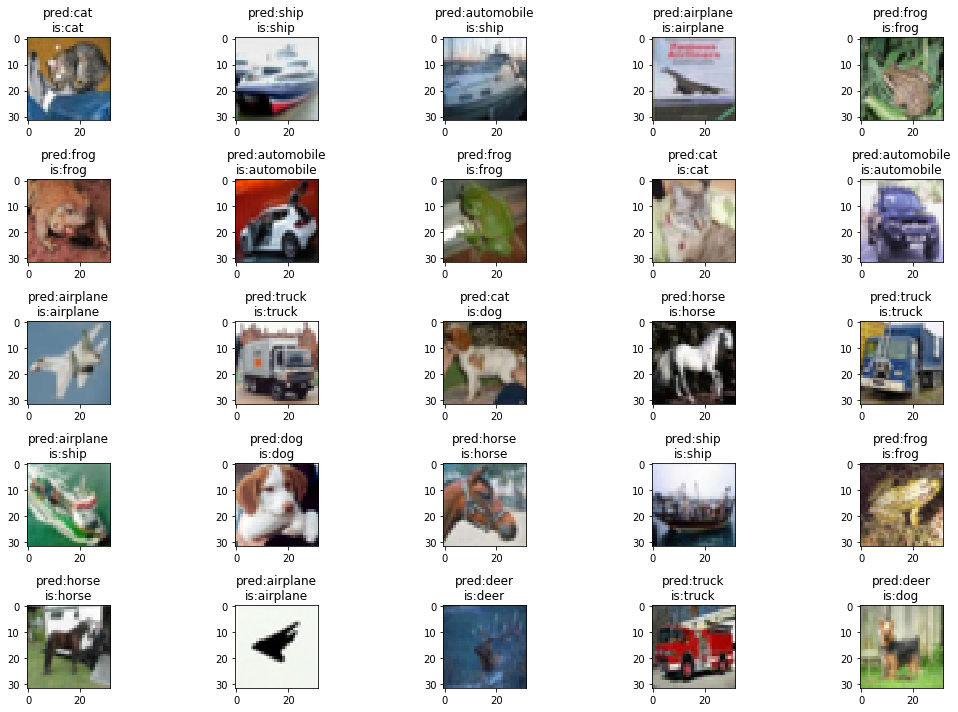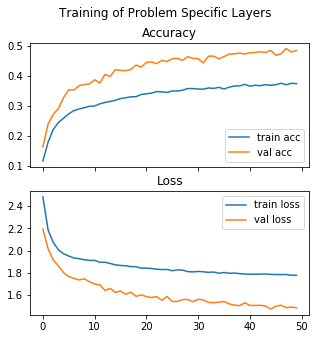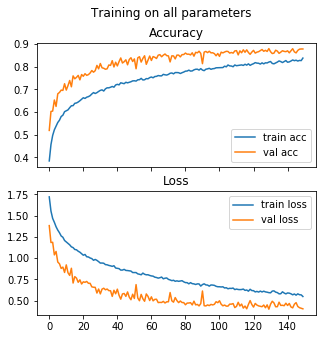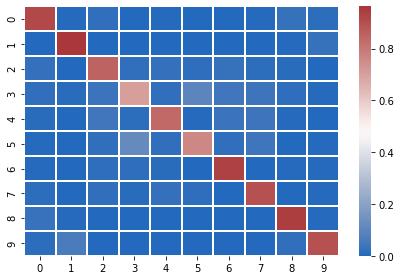# Machine Learning Final Project

This semester, I took a machine learning and pattern recognition course. The final project for this course was an exploration of deep learning on the MNIST dataset, and an accuracy competition on the CIFAR10 dataset. This notebook goes through the entire final project assignment, however, this post will only go through the final transfer learning solution I submitted for the CIFAR10 competition.Twenty Five Example Classifications

# CIFAR10

The CIFAR10 dataset consists of 60000 32x32 RGB images. Each of these images belongs to one of ten classes, with an evenly divided 6000 images per class. Additionally, 10000 of these samples are held out for cross validation.

# Transfer Learning

The intuition for transfer learning is that we can leverage knowledge gained through learning one task to more effectively and/or quickly learn another task. In practice, this is done by using the weights of a network trained on a different task as a starting point for training a new network. For this project I used the VGG19 network trained to classify ImageNet data. I chose this model because it ships with tensorflow and does not require upsampling the 32x32 images to a greater resolution. Below is the code that defines the model:

``````transfer_model=VGG19(input_shape=(32,32,3), weights='imagenet', include_top=False)

# set layers to non-trainable
for l in transfer_model.layers:
l.trainable = False

x = transfer_model.output
x = Flatten()(x)

x = Dense(1024,activation='relu')(x)
x = Dropout(0.25)(x)
x = Dense(512,activation='relu')(x)
x = Dropout(0.25)(x)
x = BatchNormalization()(x)
x = Dense(512,activation='relu')(x)
x = Dropout(0.25)(x)
x = Dense(256,activation='relu')(x)
x = Dropout(0.25)(x)
x = BatchNormalization()(x)
x = Dense(256,activation='relu')(x)
x = Dropout(0.25)(x)
x = Dense(128,activation='relu')(x)
x = Dropout(0.25)(x)
x = BatchNormalization()(x)
x = Dense(128,activation='relu')(x)
x = Dropout(0.25)(x)
x = Dense(64,activation='relu')(x)
x = Dropout(0.25)(x)
x = BatchNormalization()(x)
x = Dense(64,activation='relu')(x)
x = Dropout(0.25)(x)
x = Dense(64,activation='relu')(x)
x = Dropout(0.25)(x)
x = BatchNormalization()(x)
out = Dense(NUM_CLASSES, activation='softmax')(x)

opt_model = Model(inputs=transfer_model.inputs, outputs=out)
opt_model.compile(
loss=tf.keras.losses.categorical_crossentropy,
metrics=["accuracy", "mse"],
)``````

The first round of training only trains the layers that we added to the end of the VGG19 network. We do this so that the training of the model specific layers doesn’t degrade the quality of the weights in the pretrained network. This training last for 50 epochs:Loss and Accuracy Training on CIFAR10 Specific Layer Parameters

We then reset all layers to be trainable, and recompile the model with a lower learning rate. Then the entire model is retrained for 150 epochs, until the validation loss stopped decreasing.

``````for l in opt_model.layers:
l.trainable = True

opt_model.compile(
loss=tf.keras.losses.categorical_crossentropy,
metrics=["accuracy", "mse"],
)``````Loss and Accuracy Training on All Parameters

The visualization below give us further insight into the sources of error in the model. We can see in the confusion matrix below that classes three and five (cat and dog respectively) are getting being misclassified as the other.Confusion Matrix Across the Ten Classes

I was able to achieve a final accuracy of `87.36%` on the validation data, placing my model in the top five of the class.